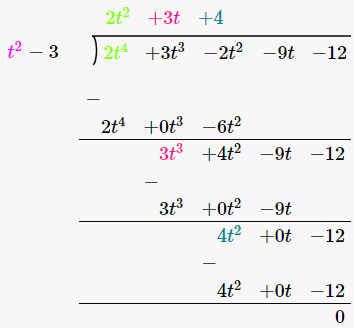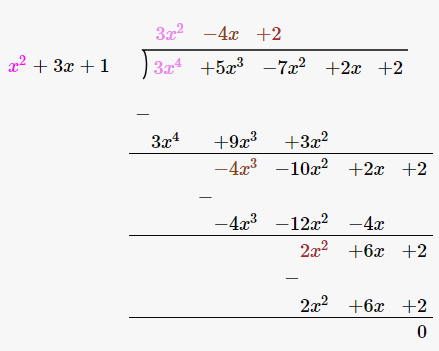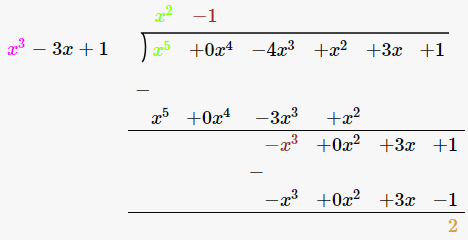# Check whether the first polynomial is a factor of the second polynomial by dividing the second polynomial by the first polynomial: (i) t2-3, 2t4 + 3t3-2t2-9t-12 (ii) x2 + 3x + 1 , 3x4 + 5x3-7x2 + 2x + 2 (iii) x2-3x + 1, x5-4x3 + x2 + 3x + 1

(i) t2-3, 2t4 +3t3-2t2-9t-12 S

As per the given data

First polynomial = t2-3

Second polynomial = 2t4 +3t3-2t2 -9t-12We observe that, the remainder is left as 0. Hence t2-3 is a factor of 2t2+3t+4.

(ii)x2+3x+1 , 3x4+5x3-7x2+2x+2

As per the given data First polynomial = x2+3x+1

Second polynomial = 3x4+5x3-7x2+2x+2We observe that, the remainder is left as 0. Hence x2 + 3x + 1 is a factor of 3x4+5x3-7x2+2x+2.

(iii) x3-3x+1, x5-4x3+x2+3x+1

As per the given data

First polynomial = x3-3x+1

Second polynomial = x5-4x3+x2+3x+1We observe that, the remainder is not equal to 0. Hence x3-3x+1 is not a factor of x5-4x3+x2+3x+1 .